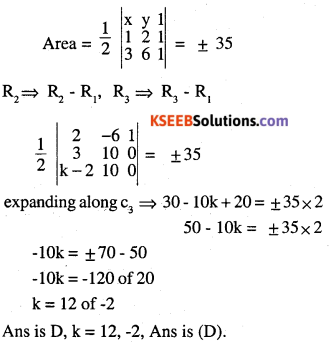# 2nd PUC Maths Question Bank Chapter 4 Determinants Ex 4.3

Students can Download Maths Chapter 4 Determinants Ex 4.3 Questions and Answers, Notes Pdf, 2nd PUC Maths Question Bank with Answers helps you to revise the complete Karnataka State Board Syllabus and score more marks in your examinations.

## Karnataka 2nd PUC Maths Question Bank Chapter 4 Determinants Ex 4.3

### 2nd PUC Maths Determinants NCERT Text Book Questions and Answers Ex 4.3

Find the area of triangle with vertices at the point given in each of the following.

Question 1.
(i) (1, 0), (6, 0), (4, 3)
Answer: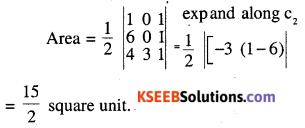(ii) (2,7)(1,1),(10,8)
Answer: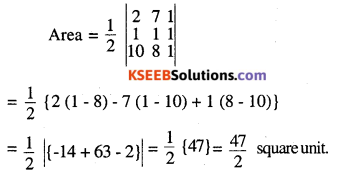(iii) (-2, -3), (3, 2), (-1, -8)
Answer: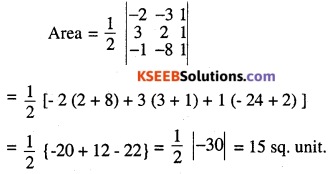Question 2.
Show that points
A (a, b + c), B (b, c + a), C (c, a + b) are collinear
Answer: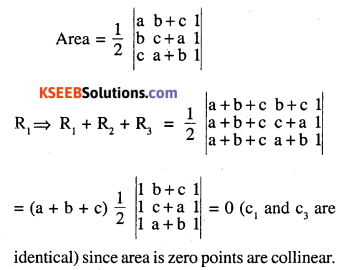Question 3.
Find the values of K if area of triangle is 4 square units and vertices are
(i) (K, 0), (4, 0), (0, 2)
Answer: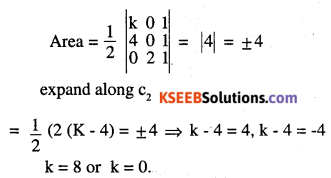(ii) (-2, 0), (0, 4), (0, k)
Answer: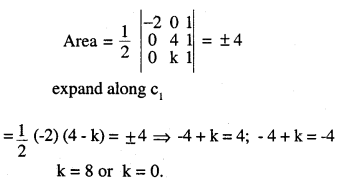Question 4.
(i) Find the equation of line joining (1, 2) and (3, 6) using determinants Let p (x, y) be any point on the line joining. (1, 2) (3, 6)
Answer: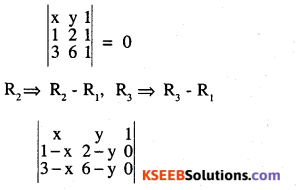expanding along
C3 ⇒1 ((1 – x) (6 – y) – (3 – x) (2 – y)) = 0
⇒ {6 – y – 6x + xy – 6 + 3y + 2x – xy}
⇒ 2y – 4x = 0, or y = 2x(ii) Find the equation of the line joining (3, 1) and (9, 3) using determinants.
Answer:
Let p (x, y) be any point on the line joining (3,1) and (9,3)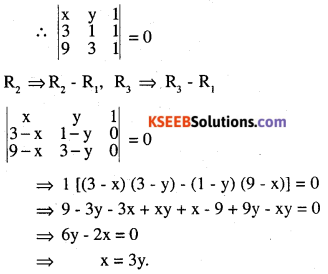Question 5.
If area of triangle is 35 square units with vertices (2, -6), (5, 4) and (k, 4) then K is
(A) 12
(B) -2
(C) -12, -2
(D) 12, -2
Answer: